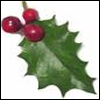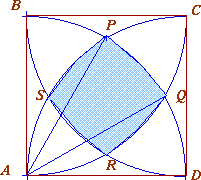#### You may also like### Giant Holly Leaf

Find the perimeter and area of a holly leaf that will not lie flat (it has negative curvature with 'circles' having circumference greater than 2πr).Given a square ABCD of sides 10 cm, and using the corners as centres, construct four quadrants with radius 10 cm each inside the square. The four arcs intersect at P, Q, R and S. Find the area enclosed by PQRS.### Get Cross

A white cross is placed symmetrically in a red disc with the central square of side length sqrt 2 and the arms of the cross of length 1 unit. What is the area of the disc still showing?

# Bound to Be

##### Age 14 to 16Challenge Level

This problem attracted several correct answers anonymously submitted by students at Madras College . This solution is included because of its brevity:$AD = 1$ unit, which is the radius of the circle centre $A$. The coordinates of $P$ are $(AD \cos 60^{\circ} , AD \sin 60^{\circ} ) = ({1\over2}, {\sqrt{3}\over2})$ Similarly coordinates of $Q$ are $(AD \cos 30^{\circ} , AD \sin 30^{\circ} ) = ( {\sqrt{3}\over2},{1\over2})$ and angle $PAQ$ is $30^{\circ}$. Area of square $PQRS = ({1\over2} - {\sqrt{3}\over2})^2 + ({\sqrt{3}\over2} - {1\over2})^2 = 2 - \sqrt{3}$ Area of a segment $PQ$ = [${1\over12}$ area of circle, centre $A$ radius $1$ unit] - [Area of Triangle $APQ$ ] Using the formula ${1\over12}\pi r^2$ for the $30$ degree sector and ${1\over2} ab\sin C$ for the area of the triangle we get: Area of a segment $PQ = {1\over12} \pi.1.1 - {1\over2}.1.1.{1\over2} = {\pi\over12} - {1\over4}$ \par Bounded area = area of square $PQRS$ + area of 4 equal segments Bounded area $= 2 - \sqrt{3} + 4 ({\pi\over12} - {1\over4}) = 1 + {\pi\over3} - \sqrt{3}$